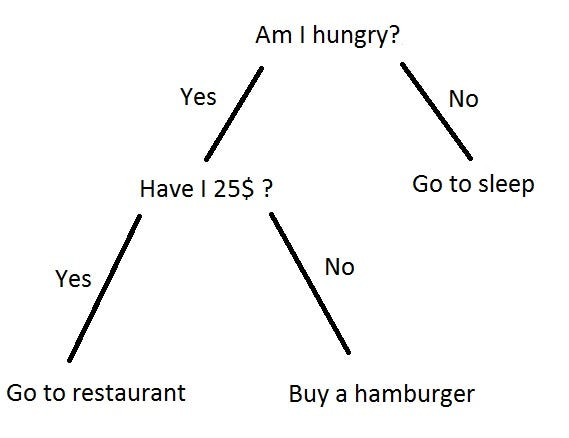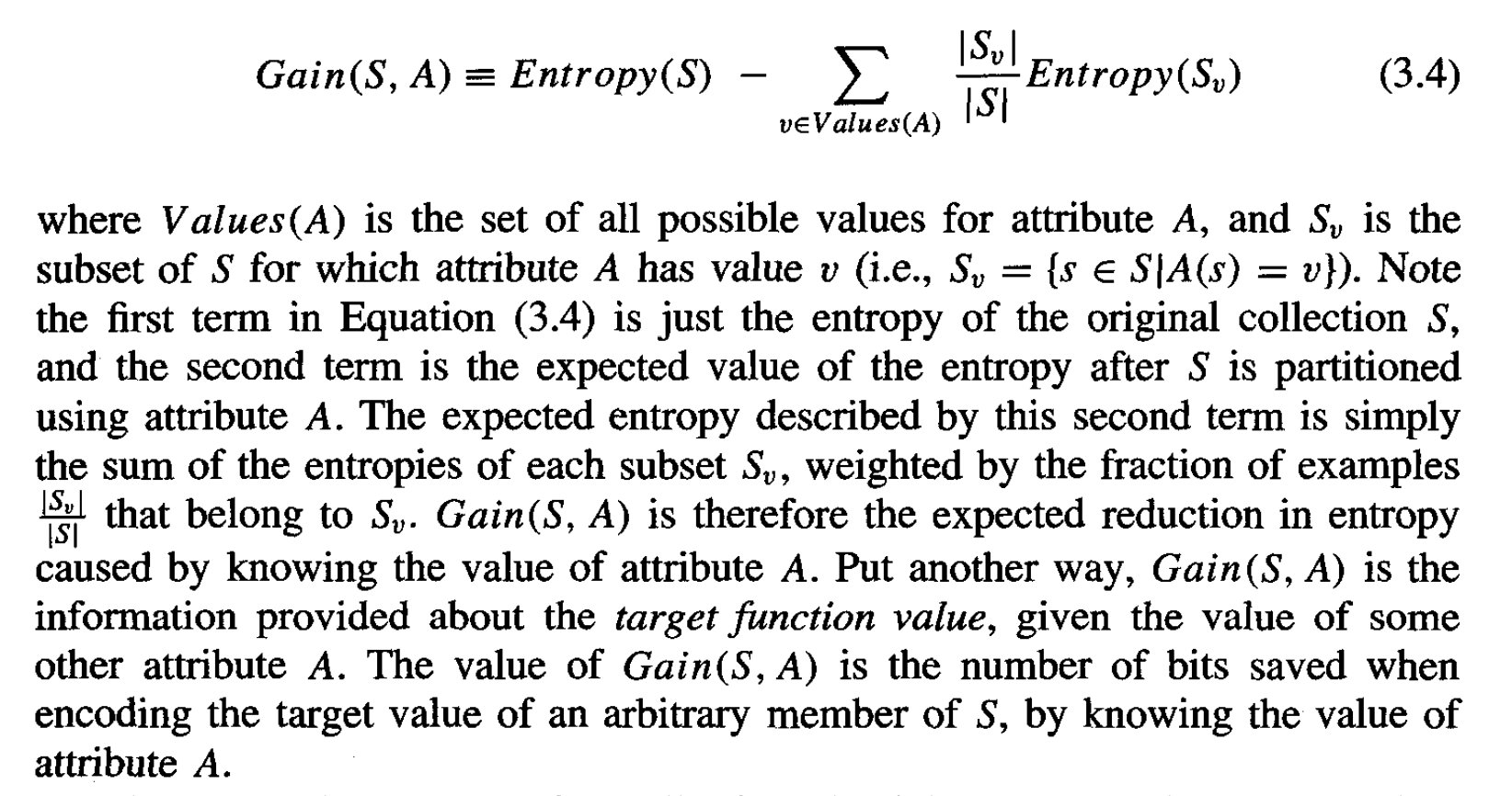# Supervised Learning: Classification Learning & Decision Trees

This is the 2nd in a series of class notes as I go through the Georgia Tech/Udacity Machine Learning course. The class textbook is Machine Learning by Tom Mitchell.

## Classification Learning

Here are some useful terms to define in classification:

• Instances (or input): values that define whatever your input space is, e.g. the pixels that make up your picture, credit score, other raw data values
• Concept: a function that actually maps inputs to outputs (or mapping an input space to a defined output space), taking Instances to some kind of output like True or False. You can also view them as defining membership in a set, e.g. “Hotdog” vs “Not Hotdog”
• Target Concept: The Concept that we are trying to find - the “actual answer”.
• Hypothesis class: The set of all Concepts (aka all classification functions) you’re willing to consider
• Sample (or Training Set): The set of all Inputs paired with a label that is a correct output.
• Candidate: Concept that you think might be the Target Concept
• Testing Set: Looks like the Training Set, but is what you test the Candidate Concept on. They should not be the same as the Training Set, because then you will not have shown the ability to generalize.

Just like in school, we test the student’s understanding of the concept by giving them new questions they haven’t seen before. If they can solve those too, then they are deemed to have learned the Target Concept.

## Decision Trees: Representation

A decision tree looks like any tree you see in computer science:Each decision node represents a question (“Am I hungry? Have I \$25?”) about an attribute, and the edges that result are complete answers to the question (here we show a binary Yes/No, but you can generalize to more discrete values). The final result is an output, where you actually decide to take an action.

## Decision Trees: Learning

Decision trees are very expressive because of their branching factor. This is illustrated in the famous game Twenty Questions. Your goal in asking each question is to narrow down the space of possibilities. So asking more general questions first is the optimal algorithm here, and we will learn how to define this now.

Here is the best algorithm to solve Twenty Questions:

``````1. Pick the best attribute to split the tree on
- ("Best" = splits the possible decision space exactly in half)
4. Go to 1. until you have an **output** (or answer, in the case of the Twenty Questions game)``````

That is almost like what we need for generalized decision trees.

## The ID3 Algorithm

The Iterative Dichotomiser 3 algorithm is a top down learning algorithm invented to programmatically generate a decision tree from a dataset. The rough pseudocode goes:

``````Loop:
Select best attribute and assign to A
Assign A as decision attribute for Node
Foreach value of A
create a descendant of Node (aka Leaf)
Sort training examples to Leaves
If examples are perfectly classified: break;
Else Iterate over Leaves``````

If you’re paying attention you’ll see that some of these steps are a lot more complicated than the others. The biggest one is “selecting the best attribute”. That is done by selecting the attribute with the highest “Gain”.

Entropy

This term is usually used in thermodynamics, but is (confusingly) also used in Information Theory. We refer here to Shannon entropy, “a measure of the unpredictability or information content of a message source”.The intuition is more important than the exact formula, and you can refer to the chart form in a binary situation:This is to say, if we were given a data set of all hotdogs, it would have zero entropy since it is already fully sorted with respect to our caring about hotdogs. Likewise, if it were all not hotdogs, it would also have zero entropy. But if it were equally mixed, we would have to start asking questions about the essential nature of a hotdog in order to try to subdivide our dataset in useful ways.

Information Gain

Information Gain is thus the decrease in total Entropy before-and-after applying a particular classification attribute:Thus, ID3 relies on iterating through and calculating each attribute’s Gain after each step, and picking the biggest Gain as the “best” attribute to split on.

There is a fuller explanation in this PDF.

## ID3 Bias

There are two forms of bias (not in the negative sense!) inherent in ID3.

• Restriction Bias - the set of all possible Hypotheses we will consider. In the case of decision trees, we’ve already decided to rule out all continuous functions.
• Preference Bias - what kinds of hypotheses from the Hypothesis set we prefer. This is at the heart of the Problem of Induction we discussed in the previous article.

ID3 biases towards “good splits at the top” and “shorter trees rather than longer trees”.

## Decision Trees: Challenges

• Continuous Attributes: How do you expand your model to handle these if they do come up? You can deal with continuous attributes by establishing cutoffs, for example Group A being Age <10, Group B being 10 <= age < 20, etc, but these categories are arbitrary and may not be useful without cheating and looking at your Testing Set.
• Scaling: You can represent all boolean combinations of attributes with decision trees: AND, OR, XOR, and so on. However the number of nodes scales exponentially - `O(2^N)` to be exact - so to make any decision at all you have to ask and answer a lot of questions. This is a problem for decision tree models.
• Stopping/Noise: Since you can repeat attributes, do you get stuck in an infinite loop if your data has some noise? (some invalid or inconsistent labelling)
• Overfitting: too large of a tree for the problem. You can mitigate by checking on a cross validation set. If an error gets low enough, you stop expanding the tree (expanding breadth first is important here). You can also try to prune trees after arriving at your final tree.

## Lazy Decision Trees

Normal Decision Trees are eager, meaning all the calculation is done upfront. A better way to go about this may be to use Lazy Decision Trees, which construct hypotheses on the fly tailored to a particular given example. You are trading off prediction time for training time here, but the gains may be disproportionate because you may pick up on definitive, fragmented features earlier (see the paper for details).

## Next in our series

Hopefully this has been a good high level overview of Decision Trees. I am planning more primers and would love your feedback and questions on:

• Overview
• Supervised Learning
• Unsupervised Learning
• Reinforcement Learning
• Markov Decision Processes - week of Mar 25
• “True” RL - week of Apr 1
• Game Theory - week of Apr 15

Tagged in:

Leave a reaction if you liked this post! 🧡